# Multi-Level Lossy Inductor (Version 8.0+)

The Multi-Level Lossy Inductor has two levels: 0 and 1. Level zero models an inductor with parallel shunt resistance. Level one adds Equivalent Series Resistance (ESR) to the model. This inductor is available with Version 8.0 or later.

The lossy inductor has a parasitic shunt resistance in parallel with the inductor. This resistance is present in both model levels and limits the high frequency response of the inductor, which in turn speeds up simulations.

In this topic:

 Model Name: Multi-Level Lossy Inductor Simulator: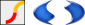This device is compatible with both the SIMetrix and SIMPLIS simulators. Parts Selector Menu Location (SIMetrix): Passives > Inductors > Multi-Level Lossy Inductor (V8.0+) Parts Selector Menu Location (SIMPLIS): Magnetics > Multi-Level Lossy Inductor (Version 8.0+) Symbol Library: passives.sxslb Model File (SIMetrix): preproc.lb Model File (SIMPLIS): simplis_param.lb Subcircuit Name: MULTI_LEVEL_LOSSY_IND_QTY Symbol: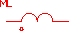Multiple Selections: Multiple devices can be selected and edited simultaneously.

## Editing the Multi-Level Lossy Inductor

To configure the Multi-Level Inductor, follow these steps:

1. Double click the symbol on the schematic to open the editing dialog to the Parameters tab.
2. Choose a model level, and then make the appropriate changes to the fields described in the table below the image.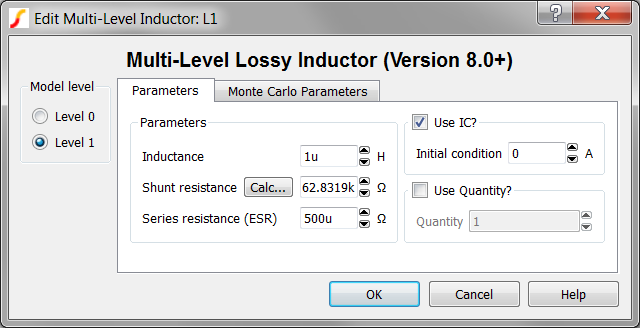Label Applicable Model(s) Units Description Inductance 0,1 H Inductance value Shunt resistance 0,1 Ω The shunt resistance in parallel with the inductor. This resistance is included to limit the maximum frequency response of the inductor, which maximizes the simulation speed. Click Calc... to calculate this value. Series resistance (ESR) 1 Ω The equivalent series resistance (ESR) Use IC? 0,1 n/a If checked, the model uses the specified Initial condition. If unchecked, the inductor is a short circuit at time=0. Initial condition 0,1 A Initial current at time=0 Use Quantity? 0,1 n/a If checked, the model uses the specified Quantity of inductors in parallel. Quantity 0,1 n/a Number of inductors in parallel. Note: To maximize simulation speed when using SIMPLIS, place a single symbol and specify a value for this parameter instead of placing multiple capacitors.

The Monte Carlo Parameters are shown and explained below.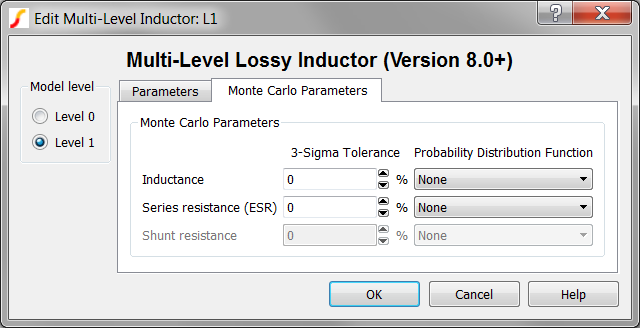Label Applicable Model(s) Units Description Inductance 0,1 % 3-sigma tolerance for the inductance value Series resistance (ESR) 1 % 3-sigma tolerance for the equivalent series resistance (ESR) Probability Distribution Function n/a n/a The choices for this function for each available parameter are the following: None Gauss Truncated Gauss Uniform Worst Case

## Multi-Level Lossy Inductor Model Levels

The Multi-Level Lossy Inductor has two levels: 0 and 1. Level zero models an inductor with parallel shunt resistance. Level 1 adds Equivalent Series Resistance (ESR) to the model.

### Level 0 Model

Level 0 models an inductor with a parallel shunt resistance.

Level 0 models these circuit elements Level 0 Schematic
 L_IND Inductance R_SHUNT Inductor shunt resistor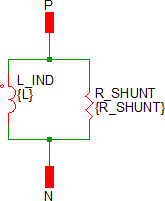### Level 1 Model

Level 1 models an inductor with a parallel shunt resistance and an equivalent series resistance (ESR).

Level 1 models these circuit elements Level 1 Schematic
 L_IND Inductance R_SHUNT Inductor shunt resistor ESR Series resistance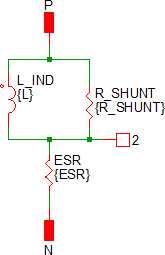## Quantity Implementation

### SIMPLIS Mode

The Multi-Level Inductor model has two quantity parameters, USE_QTY and QTY, which specify the number of inductors in parallel. Configuring these parameters minimizes the number of reactive circuit elements in the model and, therefore, provides a maximum simulation speed.

The implementation of the quantity parameter uses a "DC Transformer" technique where the inductor's terminal current is multiplied by a constant using a Current Controlled Current Source (CCCS). This technique effectively divides all resistance and inductance values by the quantity parameter (QTY). This circuitry is added if the USE_QTY parameter is set to 1.

A schematic of the quantity implementation for a Level 0 Multi-Level Inductor is shown below: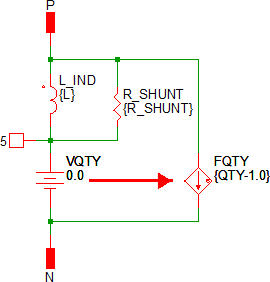### SIMetrix Mode

In SIMetrix, the quantity parameter is implemented with the "M" parameter which is common to the primitive SPICE components. This improves SIMetrix simulation speed and correctly scales resistances for noise analysis.

## Symbol Migration

Symbols placed on schematics in previous versions of SIMetrix/SIMPLIS can be automatically migrated to use the new symbols. The schematic tools menu Tools > Migrate Schematic Symbols to Version 8.20 will invoke a routine which migrates the existing symbols to the new symbols. As this action makes substantial changes to the schematic, it is recommended that you save a backup copy of the schematic first.